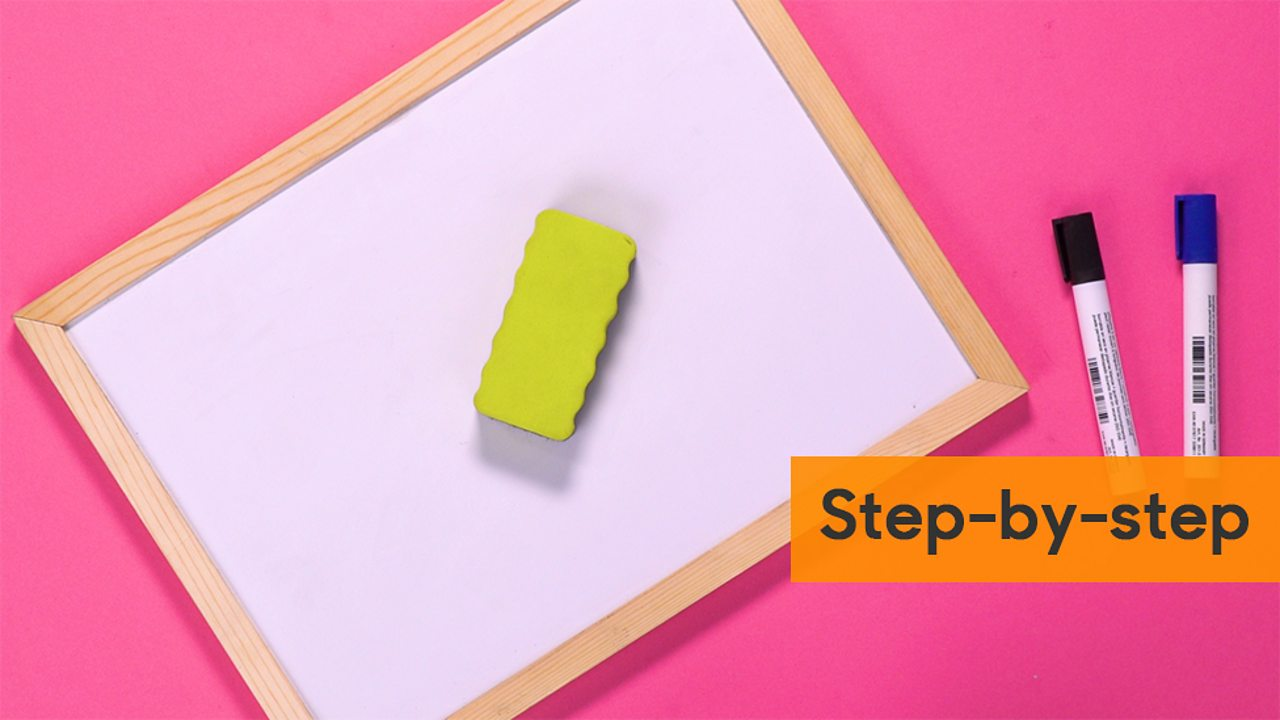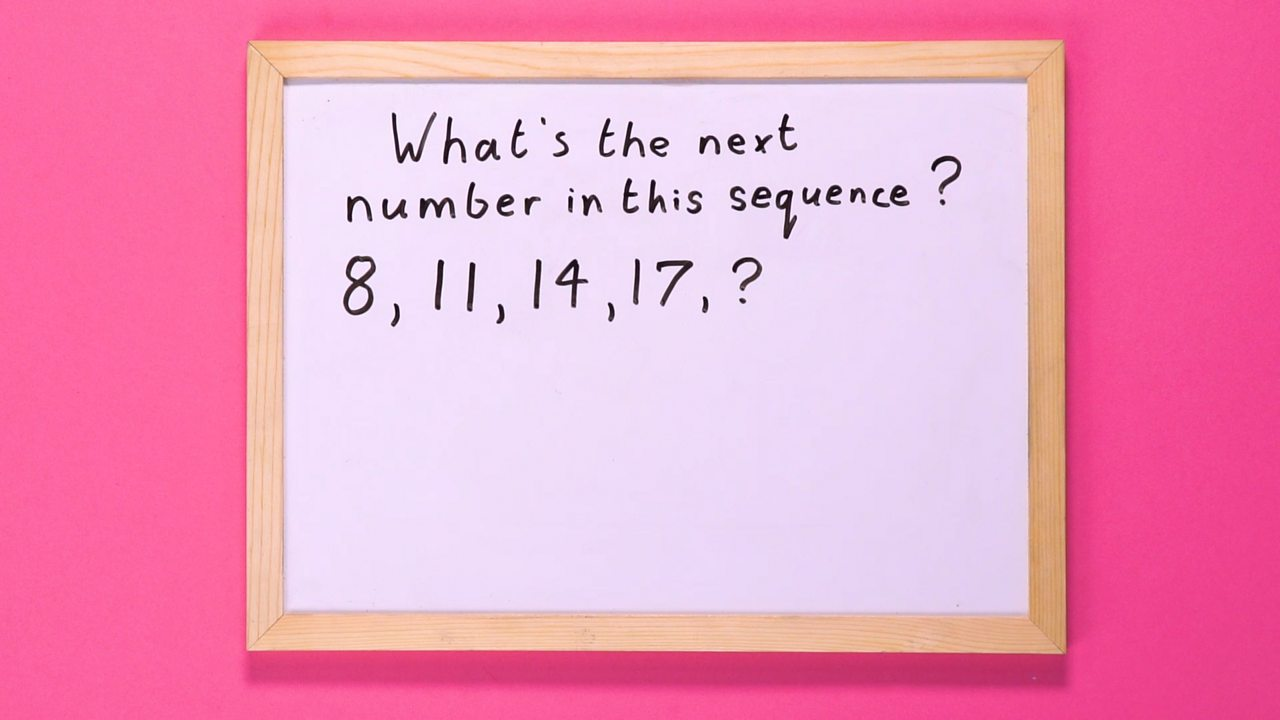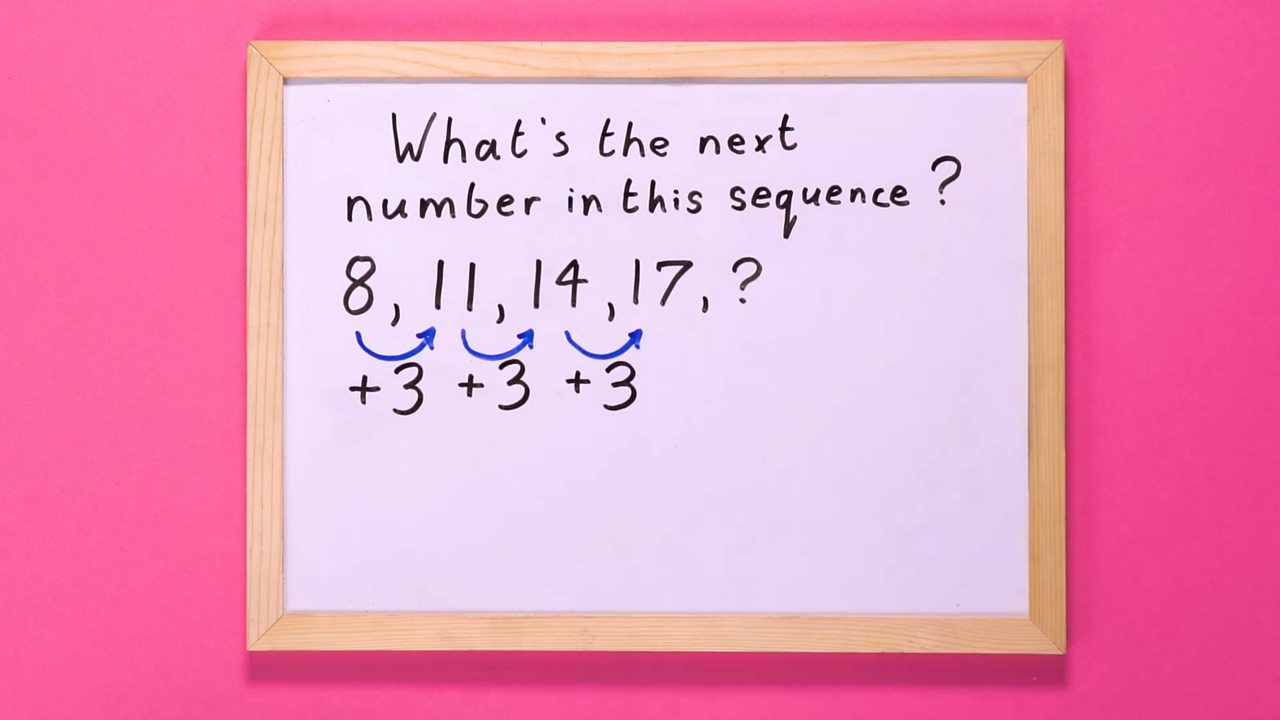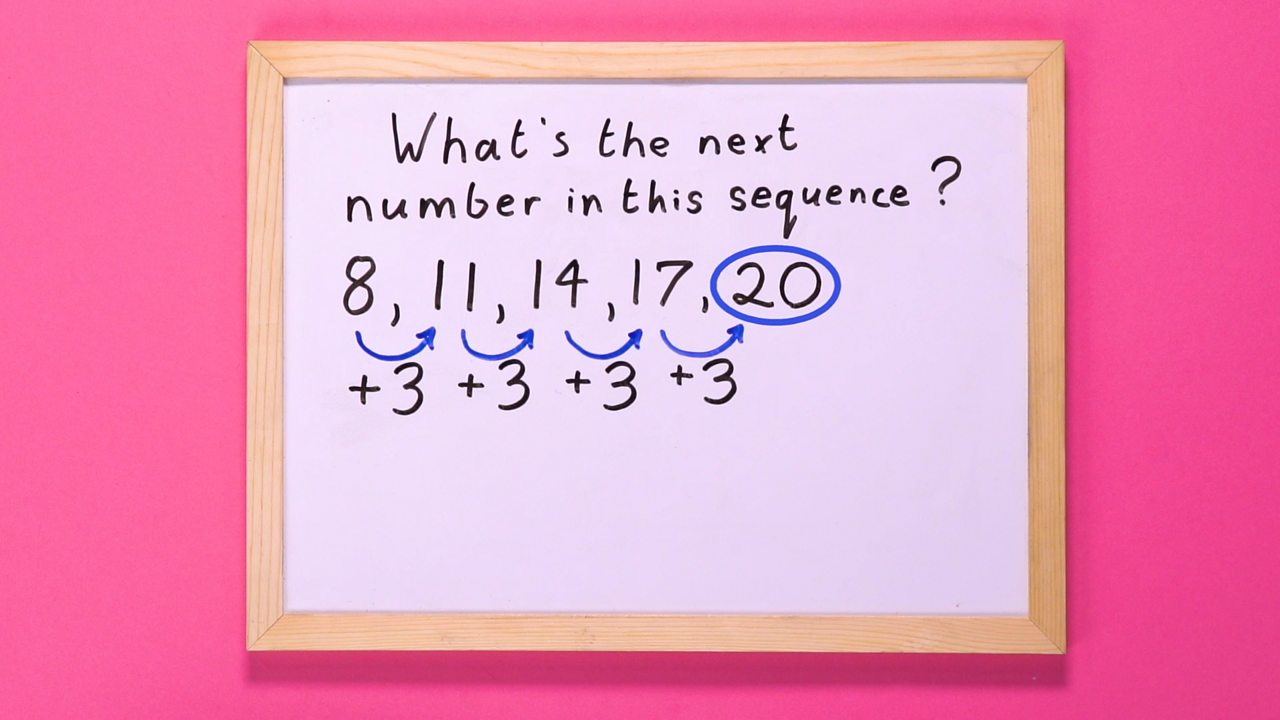# How to find the next term in an arithmetic sequence

• An arithmetic sequence goes from one term to the next by always adding (or subtracting) the same value.

• The number added (or subtracted) at each stage of the arithmetic sequence is called the common difference.

• Examples of arithmetic sequences occur when things change by the same amount each time.

## Have a goClick to see a step-by-step slideshow.1 of 5YOU WILL NEED: A whiteboard, whiteboard eraser and two coloured markers.2 of 5STEP 1: Write out the sequence.3 of 5STEP 2: Look for the common difference and write it down.4 of 5STEP 3: Use the common difference to find the next number in the sequence.5 of 5

## How do they work?

### Example 1

Here is an arithmetic sequence:

8, 11, 14, 17...

• To find the next term in this sequence we calculate the common difference.
• In this example the common difference is +3.

8 (+3) 11 (+3) 14 (+3) 17 (+3) …

Therefore, the next term in the sequence would be 20 (17 + 3)

### Example 2

Here is another arithmetic sequence:

70, 60, 50, 40 …

In this example the common difference is -10, because 10 is subtracted from each term to give the next term in the sequence.

70 (-10) 60 (-10) 50 (-10) 40 (-10) …

The next term in the sequence will be 30 (40 – 10)

When an arithmetic sequence is plotted on a graph, it always gives a straight line.

## Common examples of arithmetic sequences

• Shape patterns (see image).
• Someone walking the same distance every 10 minutes.

## Arithmetic sequence activity

Find out how much you know in this quick activity.

## Arithmetic sequence quiz

Test your knowledge with this quick quiz.